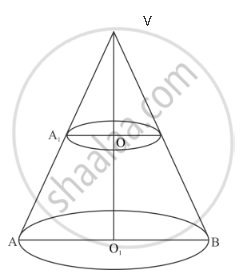Share

# The Height of a Cone is 20 Cm. a Small Cone is Cut off from the Top by a Plane Parallel to the Base. If Its Volume Be 1/125 of the Volume of the Original Cone, Determine at What Height Above the Base the Section is Made. - Mathematics

Course

#### Question

The height of a cone is 20 cm. A small cone is cut off from the top by a plane parallel to the base. If its volume be 1/125 of the volume of the original cone, determine at what height above the base the section is made.

#### Solution

We have the following situation as shown in the figureLet VAB be a cone of height h1 = VO1 =20cm. Then from the symmetric triangles VO 1A and VOA1, we have

(VO_1)/(VO)=(O_1A)/(OA_1)

⇒20/(VO)=(O_1A)/(OA_1)

It is given that, volume of the cone VA1O is1/125times the volume of the cone VAB. Hence, we have

1/3piOA_1^2xxVO=1/125xx1/3piO_1A^2xx20

⇒((OA_1)/(O_1A))^2xxVO=4/25

⇒((VO)/20)^2xxVO=4/25

⇒VO^3=(400xx4)/25

⇒ VO3 = 16 x 4

⇒ VO = 4

Hence, the height at which the section is made is 20 − 4 = 16 cm.

Is there an error in this question or solution?

#### APPEARS IN

RD Sharma Solution for Class 10 Maths (2018 (Latest))
Chapter 14: Surface Areas and Volumes
Ex. 14.3 | Q: 6 | Page no. 78
Solution The Height of a Cone is 20 Cm. a Small Cone is Cut off from the Top by a Plane Parallel to the Base. If Its Volume Be 1/125 of the Volume of the Original Cone, Determine at What Height Above the Base the Section is Made. Concept: Volume of a Combination of Solids.
S